# arvkevi / kneed

Knee point detection in Python 📈
Python Shell

## Latest commit

Latest commit 76652ce Feb 12, 2020

## Files

Type Name Latest commit message Commit time
Failed to load latest commit information..github/workflows Nov 27, 2019images Oct 1, 2019kneed Feb 13, 2020notebooks Sep 28, 2019tests Feb 12, 2020.gitattributes Nov 15, 2018.gitignore Sep 8, 2019.travis.yml Aug 3, 2019CONTRIBUTING.md Sep 10, 2019LICENSE Oct 5, 2018MANIFEST.in Jan 19, 2019README.md Feb 12, 2020postBuild Oct 30, 2018requirements.txt Sep 8, 2019setup.cfg Dec 11, 2017setup.py Sep 8, 2019

# kneed

Knee-point detection in Python

This repository is an attempt to implement the kneedle algorithm, published here. Given a set of `x` and `y` values, `kneed` will return the knee point of the function. The knee point is the point of maximum curvature.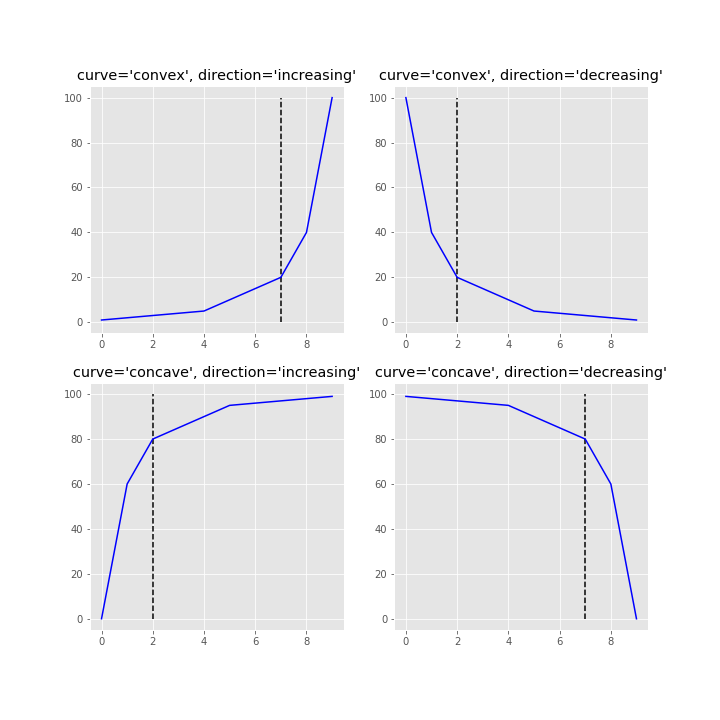## Installation

Tested with Python 3.5, 3.6, and 3.7

anaconda

`\$ conda install -c conda-forge kneed`

pip

`\$ pip install kneed`

Clone from GitHub

```\$ git clone https://github.com/arvkevi/kneed.git
\$ python setup.py install```

## Usage

These steps introduce how to use `kneed` by reproducing Figure 2 from the manuscript.

### Input Data

The `DataGenerator` class is only included as a utility to generate sample datasets.

Note: `x` and `y` must be equal length arrays.

```from kneed import DataGenerator, KneeLocator

x, y = DataGenerator.figure2()

print([round(i, 3) for i in x])
print([round(i, 3) for i in y])

[0.0, 0.111, 0.222, 0.333, 0.444, 0.556, 0.667, 0.778, 0.889, 1.0]
[-5.0, 0.263, 1.897, 2.692, 3.163, 3.475, 3.696, 3.861, 3.989, 4.091]```

### Find Knee

The knee (or elbow) point is calculated simply by instantiating the `KneeLocator` class with `x`, `y` and the appropriate `curve` and `direction`.
Here, `kneedle.knee` and/or `kneedle.elbow` store the point of maximum curvature.

```kneedle = KneeLocator(x, y, S=1.0, curve='concave', direction='increasing')

print(round(kneedle.knee, 3))
0.222

print(round(kneedle.elbow, 3))
0.222```

The knee point returned is a value along the `x` axis. The `y` value at the knee can be identified:

```print(round(kneedle.knee_y, 3))
1.897```

### Visualize

The `KneeLocator` class also has two plotting functions for quick visualizations. Note that all (x, y) are transformed for the normalized plots

```# Normalized data, normalized knee, and normalized distance curve.
kneedle.plot_knee_normalized()```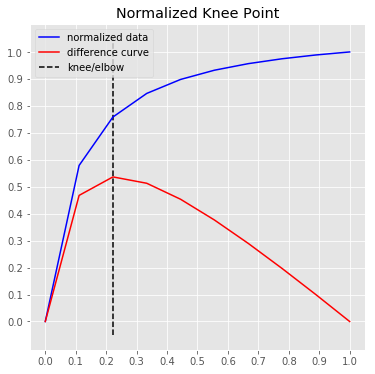```# Raw data and knee.
kneedle.plot_knee()```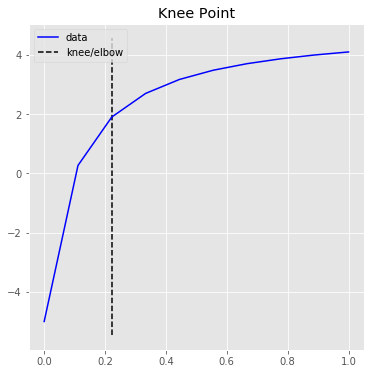## Examples

### Sensitivity Parameter (S)

The knee point selected is tunable by setting the sensitivity parameter S. From the manuscript:

The sensitivity parameter allows us to adjust how aggressive we want Kneedle to be when detecting knees. Smaller values for S detect knees quicker, while larger values are more conservative. Put simply, S is a measure of how many “flat” points we expect to see in the unmodified data curve before declaring a knee.

```import numpy as np
np.random.seed(23)

sensitivity = [1, 3, 5, 10, 100, 200, 400]
knees = []
norm_knees = []

n = 1000
x = range(1, n + 1)
y = sorted(np.random.gamma(0.5, 1.0, n), reverse=True)
for s in sensitivity:
kl = KneeLocator(x, y, curve='convex', direction='decreasing', S=s)
knees.append(kl.knee)
norm_knees.append(kl.norm_knee)

print(knees)
[43, 137, 178, 258, 305, 482, 482]

print([nk.round(2) for nk in norm_knees])
[0.04, 0.14, 0.18, 0.26, 0.3, 0.48, 0.48]

import matplotlib.pyplot as plt
plt.style.use('ggplot');
plt.figure(figsize=(8, 6));
plt.plot(kl.x_normalized, kl.y_normalized);
plt.plot(kl.x_distance, kl.y_distance);
colors = ['r', 'g', 'k', 'm', 'c', 'orange']
for k, c, s in zip(norm_knees, colors, sensitivity):
plt.vlines(k, 0, 1, linestyles='--', colors=c, label=f'S = {s}');
plt.legend();```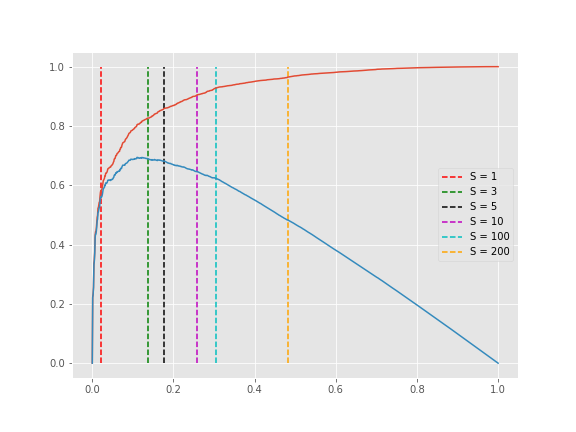Notice that any S>200 will result in a knee at 482 (0.48, normalized) in the plot above.

### Online vs Offline Detection

The knee point can be corrected if the parameter `online` is `True` (default). This mode will step through each element in `x`. In contrast, if `online` is `False`, kneed will run in offline mode and return the first knee point identified.

Using the `x` and `y` from the Sensitivity example above, this time, let's keep `S=1` but modify `online`.

```kl_online = KneeLocator(x, y, curve='convex', direction='decreasing', online=True)
kl_offline = KneeLocator(x, y, curve='convex', direction='decreasing', online=False)

import matplotlib.pyplot as plt
plt.style.use('ggplot');
plt.figure(figsize=(8, 6));
plt.plot(kl_online.x_normalized, kl_online.y_normalized);
plt.plot(kl_online.x_distance, kl_online.y_distance);
colors = ['r', 'g']
for k, c, o in zip([kl_online.norm_knee, kl_offline.norm_knee], ['r', 'g'], ['online', 'offline']):
plt.vlines(k, 0, 1, linestyles='--', colors=c, label=o);
plt.legend();```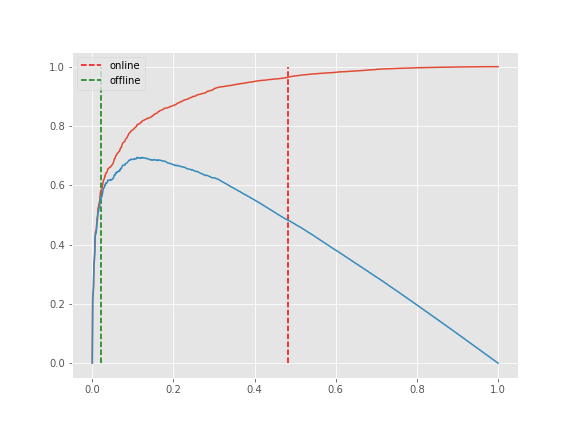### Polynomial Fit

Here is an example of a "bumpy" or "noisy" line where the default `scipy.interpolate.interp1d` spline fitting method does not provide the best estimate for the point of maximum curvature. This example demonstrates that setting the parameter `interp_method='polynomial'` will choose a more accurate point by smoothing the line.

The argument for `interp_method` parameter is a string of either "interp1d" or "polynomial".

```x = list(range(90))
y = [
7304, 6978, 6666, 6463, 6326, 6048, 6032, 5762, 5742,
5398, 5256, 5226, 5001, 4941, 4854, 4734, 4558, 4491,
4411, 4333, 4234, 4139, 4056, 4022, 3867, 3808, 3745,
3692, 3645, 3618, 3574, 3504, 3452, 3401, 3382, 3340,
3301, 3247, 3190, 3179, 3154, 3089, 3045, 2988, 2993,
2941, 2875, 2866, 2834, 2785, 2759, 2763, 2720, 2660,
2690, 2635, 2632, 2574, 2555, 2545, 2513, 2491, 2496,
2466, 2442, 2420, 2381, 2388, 2340, 2335, 2318, 2319,
2308, 2262, 2235, 2259, 2221, 2202, 2184, 2170, 2160,
2127, 2134, 2101, 2101, 2066, 2074, 2063, 2048, 2031
]

# the default spline fit, `interp_method='interp1d'`
kneedle = KneeLocator(x, y, S=1.0, curve='convex', direction='decreasing', interp_method='interp1d')
kneedle.plot_knee_normalized()```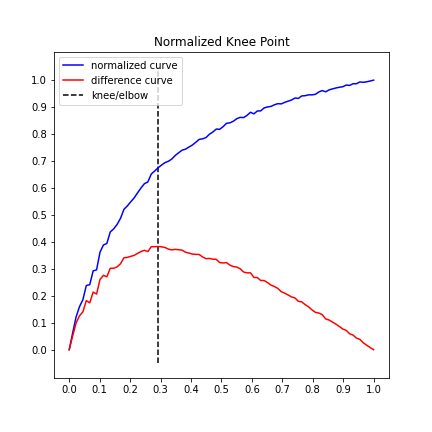```# The same data, only using a polynomial fit this time.
kneedle = KneeLocator(x, y, S=1.0, curve='convex', direction='decreasing', interp_method='polynomial')
kneedle.plot_knee_normalized()```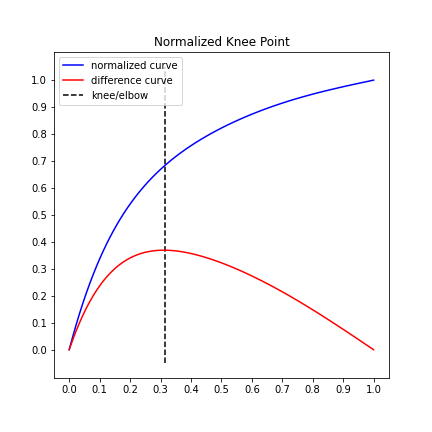### NoisyGaussian

Figure 3 from the manuscript estimates the knee to be `x=60` for a `NoisyGaussian`. This simulate 5,000 `NoisyGaussian` instances and finds the average.

```knees = []
for i in range(5):
x, y = DataGenerator.noisy_gaussian(mu=50, sigma=10, N=1000)
kneedle = KneeLocator(x, y, curve='concave', direction='increasing', interp_method='polynomial', online=True)
knees.append(kneedle.knee)

# average knee point
round(sum(knees) / len(knees), 3)
63.583```

### Select k clusters

Find the optimal number of clusters (k) to use in k-means clustering. See the tutorial in the notebooks directory.

`KneeLocator(x, y, curve='convex', direction='decreasing')`